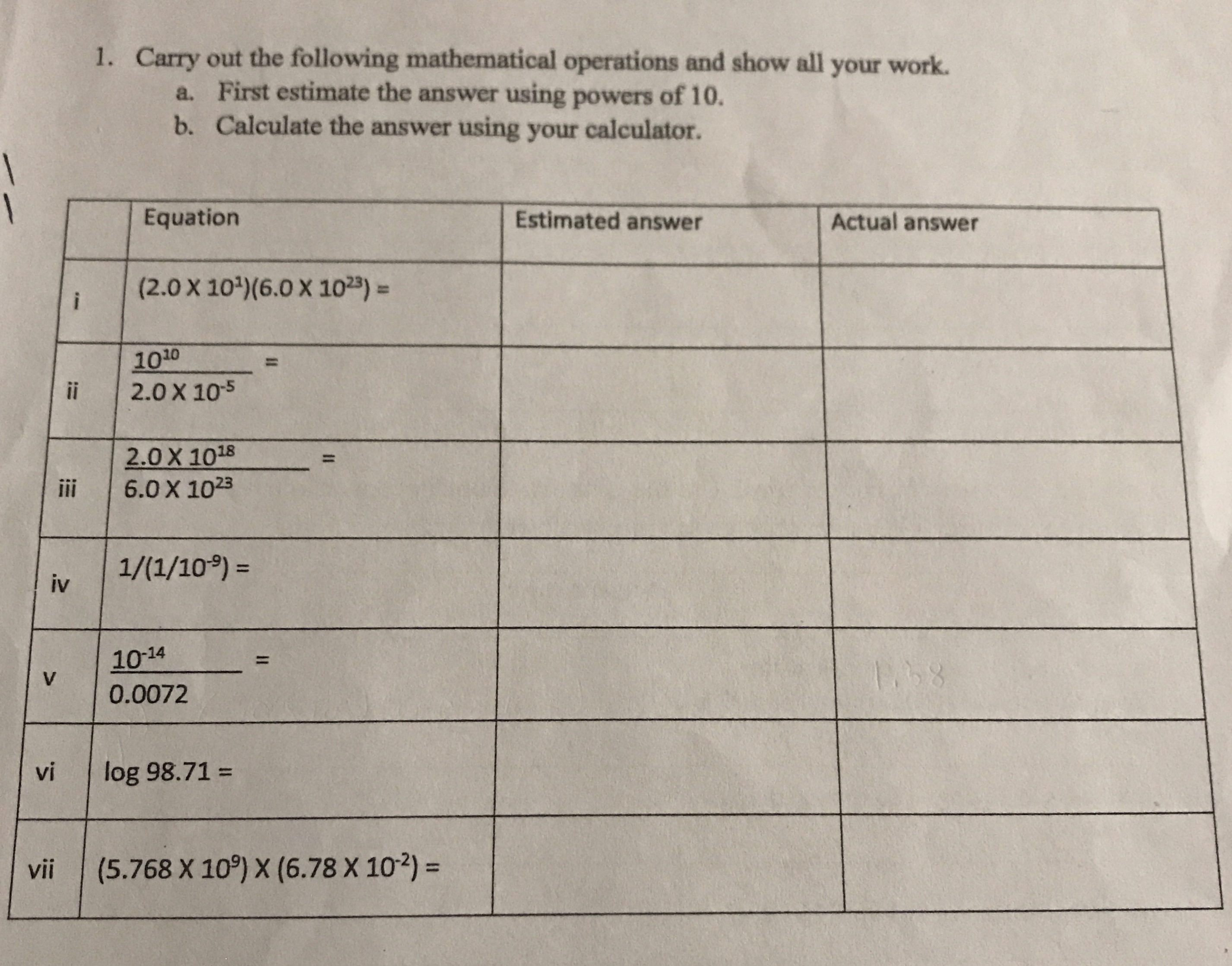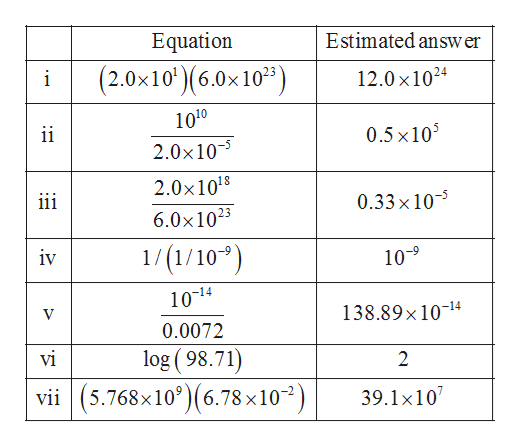# Carry out the following mathematical operations and show all your work.a. First estimate the answer using powers of 10.b. Calculate the answer using your calculator.1.1EquationEstimated answerActual answer(2.0 X 10) (6.0 X 1023)i10102.0 X 1051ii2.0 X 10186.0 X 1023ii1/(1/10)=iv10-14111.58V0.0072log 98.71=vi(5.768 X 10) X (6.78 X 102) =viiII

Question
37 viewshelp_outlineImage TranscriptioncloseCarry out the following mathematical operations and show all your work. a. First estimate the answer using powers of 10. b. Calculate the answer using your calculator. 1. 1 Equation Estimated answer Actual answer (2.0 X 10) (6.0 X 1023) i 1010 2.0 X 105 1 ii 2.0 X 1018 6.0 X 1023 ii 1/(1/10)= iv 10-14 11 1.58 V 0.0072 log 98.71= vi (5.768 X 10) X (6.78 X 102) = vii II fullscreen
check_circle

Step 1

Option (a): The estimated answers us...help_outlineImage TranscriptioncloseEquation Estimated answ er (2.0x10)(6.0x10) 12.0 x1024 1010 ii 0.5x10 2.0x105 2.0x1018 iii 0.33 x 10 6.0x1023 1/(1/109 iv 10-9 10-14 138.89x1014 0.0072 log ( 98.71) vii(5.768x10)(6.78 x10) vi 2 39.1x10 fullscreen

### Want to see the full answer?

See Solution

#### Want to see this answer and more?

Solutions are written by subject experts who are available 24/7. Questions are typically answered within 1 hour.*

See Solution
*Response times may vary by subject and question.
Tagged in

### General Chemistry